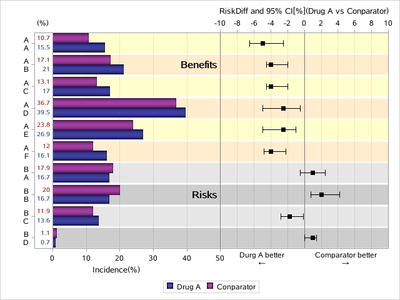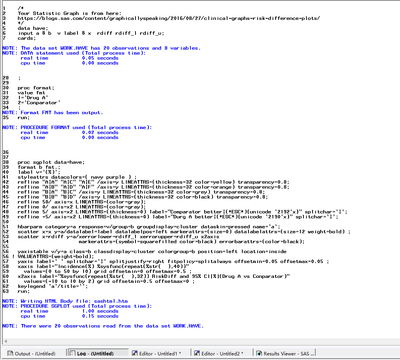## Graph with risk

Dear All,

I found a part of the program, but I can't reproduce the attached chart.
I'm not a good programmer and therefore I have some difficulty finding the solution, can I ask you for help?
Thank you

proc sgplot data=tmp2 dattrmap=AttrMap nocycleattrs sganno=anno;

/* This syntax plots the incidence lines on LHS */
highlow y=cat high=rate low=zero / group=group type=bar groupdisplay=cluster name='a'
lowlabel=IRLAB barwidth=1.0 clusterwidth=0.9 transparency=0 fill attrid=group
dataskin=pressed LABELATTRS= (size=8pt weight=normal family='Albany AMT') nooutline;

/* This syntax plots background shading */
highlow y=cat high=xmax low=rate1 / group=brtyp type=bar groupdisplay=cluster
barwidth=1.0 clusterwidth=0.9 transparency=0.70 fill attrid=brtyp nooutline;

/* This syntax plots the words Benefits and Risks at the respective y axis posotions */
highlow y=cat high=defx low=defx / type=bar highlabel=BRTXT barwidth=0 transparency=0 fill
LABELATTRS= (size=20pt weight=normal family='Albany AMT') nooutline;

/* This syntax plots the risk difference with error bars on RHS */
scatter y=cat2 x=rd /group=subg xerrorlower=rdlo xerrorupper=rdhi
x2axis y2axis attrid=subg;

/* Axis limits (0 to 50; -8 to 😎 might need to be adjusted depending on data */
refline 50 / axis=x;
refline 0 /axis=x2;

xaxis display=(nolabel) offsetmax=0.60 grid values=(0 to 50 by 10) valueattrs=(size=7);
yaxis display=(nolabel) valueattrs=(size=9 family='Albany AMT') type=discrete
fitpolicy=splitalways splitchar="#";

x2axis display=(nolabel) offsetmin=0.42 grid max=64 values=(-10 to 10 by 2) valueattrs=(size=7);
y2axis display=(nolabel noticks novalues) values=(1 to 10 by 1) type=linear;

keylegend 'a';
run;

1 ACCEPTED SOLUTION

Accepted SolutionsKsharp
Super User

## Re: Graph with risk

``````/*
Your Statistic Graph is from here:
https://blogs.sas.com/content/graphicallyspeaking/2016/08/27/clinical-graphs-risk-difference-plots/
*/
data have;
input a \$ b  v label \$ x  rdiff rdiff_l rdiff_u;
cards;
A|A 1 15.5 . . -5 -6.5 -2.5
A|A 2 10.7 . . . . .
A|B 1 21.0 Benefits 50  -4 -4.5 -2
A|B 2 17.1 . . . . .
A|C 1 17.0 . . -4 -4.5 -2
A|C 2 13.1 . . . . .
A|D 1 39.5 . . -2.5 -5 -0.5
A|D 2 36.7 . . . . .
A|E 1 26.9 . . -2.5 -5 -1
A|E 2 23.8 . . . . .
A|F 1 16.1 . . -4 -4.8 -2.2
A|F 2 12.0 . . . . .
B|A 1 16.7 . . 1 -0.5 2.5
B|A 2 17.9 . . . . .
B|B 1 16.7 . . 2 0.8 4.2
B|B 2 20.0 Risks 50 . . .
B|C 1 13.6 . . -1.8 -2.8 -0.1
B|C 2 11.9 . . . . .
B|D 1 0.7  . . 1 0 1.4
B|D 2 1.1  . . . . .
;

proc format;
value fmt
1='Drug A'
2='Conparator'
;
run;

proc sgplot data=have;
format b fmt.;
label v='(%)';
styleattrs datacolors=( navy purple ) ;
refline "A|A" "A|C" "A|E" /axis=y LINEATTRS=(thickness=32 color=yellow) transparency=0.8;
refline "A|B" "A|D" "A|F" /axis=y LINEATTRS=(thickness=32 color=orange) transparency=0.8;
refline "B|A" "B|C" /axis=y LINEATTRS=(thickness=32 color=grey) transparency=0.8;
refline "B|B" "B|D" /axis=y LINEATTRS=(thickness=32 color=black) transparency=0.8;
refline 50/ axis=x LINEATTRS=(color=gray);
refline 0/ axis=x2 LINEATTRS=(color=gray);
refline 5/ axis=x2 LINEATTRS=(thickness=0) label="Comparator better|(*ESC*){unicode '2192'x}" splitchar='|';
refline -5/ axis=x2 LINEATTRS=(thickness=0) label="Durg A better|(*ESC*){unicode '2190'x}" splitchar='|';

hbarparm category=a response=v/group=b groupdisplay=cluster dataskin=pressed name='a';
scatter x=x y=a/datalabel=label datalabelpos=left markerattrs=(size=0) datalabelattrs=(size=12 weight=bold) ;
scatter x=rdiff y=a/xerrorlower=rdiff_l xerrorupper=rdiff_u x2axis
markerattrs=(symbol=squarefilled color=black) errorbarattrs=(color=black);

yaxistable v/y=a class=b classdisplay=cluster colorgroup=b position=left location=inside VALUEATTRS=(weight=bold);
yaxis label=' ' splitchar='|' splitjustify=right fitpolicy=splitalways offsetmin=0.05 offsetmax=0.05 ;
xaxis label="Incidence(%) %sysfunc(repeat(%str(  ),40))"
values=(0 to 50 by 10) grid offsetmin=0 offsetmax=0.5 ;
x2axis label="%sysfunc(repeat(%str(  ),32)) RiskDiff and 95% CI[%](Drug A vs Conparator)"
values=(-10 to 10 by 2) grid offsetmin=0.5 offsetmax=0 ;
keylegend 'a'/title='';
run;``````6 REPLIES 6

## Re: Graph with risk

Data, can't test code without data. Also you should paste text into a text box opened on the forum with the </> icon that appears above the message window. If you look at your post some combination of characters have been turned into an emoji which is just one of the ways the forum software will reformat pasted text.

The percent column would likely be done with a YAXIS table, depending on the content of your data.

You don't say which part(s) of the graph you are having problems with so it is extremely hard to tell what changes to code may be needed. And as I mentioned, we can't test a solution without data. Often a change to the data is needed for specific plots.

Instructions here: https://communities.sas.com/t5/SAS-Communities-Library/How-to-create-a-data-step-version-of-your-dat... will show how to turn an existing SAS data set into data step code that can be pasted into a forum code box using the </> icon or attached as text to show exactly what you have and that we can test code against.

## Re: Graph with risk

Sorry if I didn't attach the data.
In practice I didn't have a database to show, I thought that the graph could be generated by generating a fancy DB.
I have attached the program that I had found on the internet and I thought it would make programming easier.
I'll be more careful next time.

GiuseppeKsharp
Super User

## Re: Graph with risk

``````/*
Your Statistic Graph is from here:
https://blogs.sas.com/content/graphicallyspeaking/2016/08/27/clinical-graphs-risk-difference-plots/
*/
data have;
input a \$ b  v label \$ x  rdiff rdiff_l rdiff_u;
cards;
A|A 1 15.5 . . -5 -6.5 -2.5
A|A 2 10.7 . . . . .
A|B 1 21.0 Benefits 50  -4 -4.5 -2
A|B 2 17.1 . . . . .
A|C 1 17.0 . . -4 -4.5 -2
A|C 2 13.1 . . . . .
A|D 1 39.5 . . -2.5 -5 -0.5
A|D 2 36.7 . . . . .
A|E 1 26.9 . . -2.5 -5 -1
A|E 2 23.8 . . . . .
A|F 1 16.1 . . -4 -4.8 -2.2
A|F 2 12.0 . . . . .
B|A 1 16.7 . . 1 -0.5 2.5
B|A 2 17.9 . . . . .
B|B 1 16.7 . . 2 0.8 4.2
B|B 2 20.0 Risks 50 . . .
B|C 1 13.6 . . -1.8 -2.8 -0.1
B|C 2 11.9 . . . . .
B|D 1 0.7  . . 1 0 1.4
B|D 2 1.1  . . . . .
;

proc format;
value fmt
1='Drug A'
2='Conparator'
;
run;

proc sgplot data=have;
format b fmt.;
label v='(%)';
styleattrs datacolors=( navy purple ) ;
refline "A|A" "A|C" "A|E" /axis=y LINEATTRS=(thickness=32 color=yellow) transparency=0.8;
refline "A|B" "A|D" "A|F" /axis=y LINEATTRS=(thickness=32 color=orange) transparency=0.8;
refline "B|A" "B|C" /axis=y LINEATTRS=(thickness=32 color=grey) transparency=0.8;
refline "B|B" "B|D" /axis=y LINEATTRS=(thickness=32 color=black) transparency=0.8;
refline 50/ axis=x LINEATTRS=(color=gray);
refline 0/ axis=x2 LINEATTRS=(color=gray);
refline 5/ axis=x2 LINEATTRS=(thickness=0) label="Comparator better|(*ESC*){unicode '2192'x}" splitchar='|';
refline -5/ axis=x2 LINEATTRS=(thickness=0) label="Durg A better|(*ESC*){unicode '2190'x}" splitchar='|';

hbarparm category=a response=v/group=b groupdisplay=cluster dataskin=pressed name='a';
scatter x=x y=a/datalabel=label datalabelpos=left markerattrs=(size=0) datalabelattrs=(size=12 weight=bold) ;
scatter x=rdiff y=a/xerrorlower=rdiff_l xerrorupper=rdiff_u x2axis
markerattrs=(symbol=squarefilled color=black) errorbarattrs=(color=black);

yaxistable v/y=a class=b classdisplay=cluster colorgroup=b position=left location=inside VALUEATTRS=(weight=bold);
yaxis label=' ' splitchar='|' splitjustify=right fitpolicy=splitalways offsetmin=0.05 offsetmax=0.05 ;
xaxis label="Incidence(%) %sysfunc(repeat(%str(  ),40))"
values=(0 to 50 by 10) grid offsetmin=0 offsetmax=0.5 ;
x2axis label="%sysfunc(repeat(%str(  ),32)) RiskDiff and 95% CI[%](Drug A vs Conparator)"
values=(-10 to 10 by 2) grid offsetmin=0.5 offsetmax=0 ;
keylegend 'a'/title='';
run;``````## Re: Graph with risk

Thanks for the program.
I tried to run it, but in the Log it gives me an error for the instruction groupdisplay=cluster and classdisplay=cluster.Ksharp
Super User

## Re: Graph with risk

What version of sas you are using ?
Mine is SAS 9.4M7 .
If your sas is too old, you could try free version:
https://welcome.oda.sas.com/

I didn't get any error info in my log.
You'd better post your full LOG.## Re: Graph with risk

Dear Ksharp,

thanks you wholeheartedly.

The problem was my SAS version which I have now updated and your program is perfect.
You have been very kind.

Giuseppe

Discussion stats
• 6 replies
• 797 views
• 5 likes
• 3 in conversation